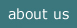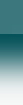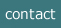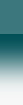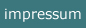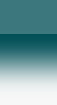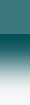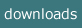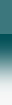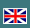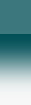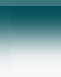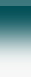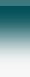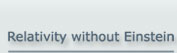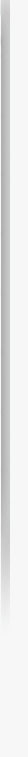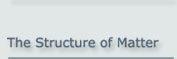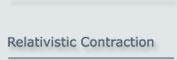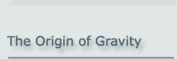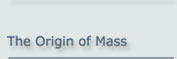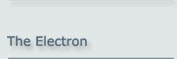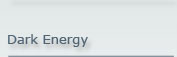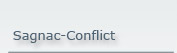Dark Energy - Really Needed?

Not needed! The apparent acceleration of recent supernovae 1a is merely
an evaluation result.

1 Summary

Dark Energy is the explanation of current physics for the apparent acceleration of the universe.

Observations of supernovae of type 1a are said to show an accelerated expansion speed of the universe at recent time. This observation is, however, questionable.

The apparent acceleration of the objects can be explained as a measurement result. Depending on the understanding of relativity in general and of gravity in special (i.e. whether on the base of Einstein’s relativity or not), there are two solutions presented, which explain why this acceleration seems to exist.

2 The Apparent Recent Acceleration

The acceleration of the objects of our universe at recent times is concluded from the observations of several teams. The result of Riess et al.  from the observation of supernovae type 1a is presented in figure 2.1.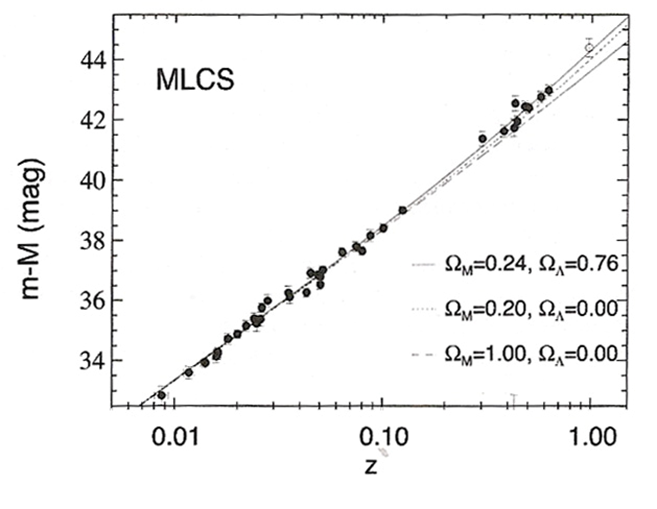Figure 2.1: Supernova 1a Hubble diagram

Figure 2.1 shows the apparent magnitude of the observed supernovae (as the ordinate) versus the red shift z (the abscissa), which is identified with the escape speed of the star.

The red shift z is defined as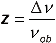(2.1)

where νob is the observed frequency, Δν is the frequency shift. From this follows: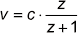(2.2)

which is used for the evaluation according to figure 2.1, but conventionally with the assumption that c is a constant over all times and the space is unchanged during the time investigated.

According to the Hubble Law, all stars and so also the investigated supernovae should be situated on a straight line, presented in figure 2.1 by the dotted line for the most probable situation / assumption. This means that the escape speed of these objects is proportional to their distance to the observer. However, the measurement points in the upper part can be understood as being too much to the left, which means that the red shift of the older supernovae is too small in comparison to the younger stars, being presented in the lower (left) part. This is commonly interpreted in the way that the younger supernovae are too fast in comparison to the older ones. They are assumed to be accelerated.

The lower - dashed - line would also be acceptable but assumes a higher matter density of the universe indicated by ΩM = 1. The lowest - straight - line which does fit to the data is characterized by ΩΛ = 1, which means the assumption of an acceleration, here indicated by the denotation of the cosmological constant Λ with reference to the original conception of Einstein.

Presently there are the following models used to explain the phenomenon:
• Dark Energy: This means that the whole universe is filled by some type of energy which causes all objects to accelerate. This energy can, with reference to Einstein’s understanding of mass and energy, identified with matter. This leads to the conclusion that – together with the mysterious Dark Matter – only ca. 4% of the matter of the universe are known and described by present physics
• Quintessence: This would be some kind of a potential which can be related to a new type of matter which in turn could be understood as some modified type of an ether
• The recreation of the cosmological constant Λ, which was once introduced by Einstein in order to explain the fact that the universe does not collapse. This idea was lapsed at some later time, after Hubble detected that the universe was in a state of permanent expansion. Einstein called this idea later his worst stupidity.

3 Solutions Related to the Idea of Inflation

For the explanation presented here it is first necessary to say some words about the so called ‘inflation’ of the universe.

3.1 The Inflation of the Universe - Why is it Needed?

From the temperature distribution of the Cold Microwave Background (CMB) it is concluded that there must have been a correlation between certain regions of the universe at a very short time after the Big Bang. On the other hand, those regions moved off each other at such a high speed that, in the face of the limited speed of light, a causal connection cannot be understood. This question was given the name “Horizon Problem”.

3.2 The Understanding of Inflation in Present Cosmology

For this causal conflict present cosmology offers a solution, which is based on a specific understanding of the spatial situation. According to this solution, the extension of the physical ‘space’ was at the start time of the universe smaller than at later times by a huge factor of around 1050. Under these circumstances a logical connection is possible. Immediately after this time, an expansion happened by just this factor. This latter phenomenon has received the name ‘inflation’. And up to now there is still some inflation (expansion) assumed, but at a much reduced rate.

This development is visualized by the commonly known figure 3.1.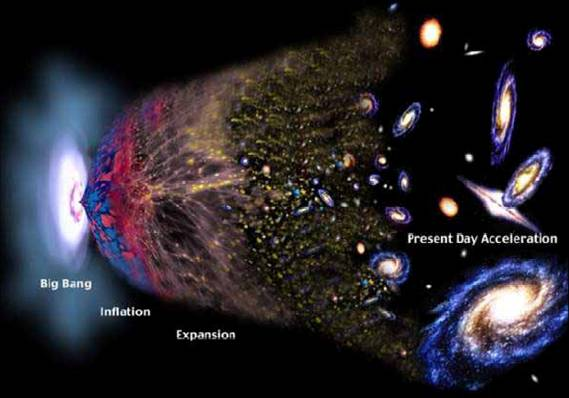Figure 3.1: The spatial expansion after the Big Bang

Presently there is no understanding what the cause of the inflation could be.

The correlation problem can, however, be solved as well with reference to the other of the two conflicting parameters, the speed of light.

3.3 The Alternative Explanation to Inflation

From a logical point, the problem of correlation is a conflict between the spatial extension and the speed of light.

So, as an alternative to an assumed change of the ‘space’, it can as well be assumed that the speed of light has changed in the way that it was extremely large in a short period after the Big Bang. Afterwards the speed of light decreased rapidly at first, and later in a slow manner until the present time as shown in figure 3.2.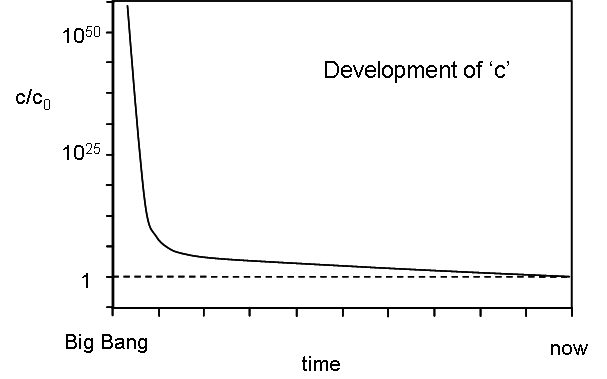Figure 3.2: Development of c after the Big Bang

The assumption that the speed of light has changed during the development of the universe is anyway attractive, as it would not only solve this causal problem. As a further benefit, it would also solve the presently not understood fine-tuning of basic physical parameters. (Refer e.g. to the work of J. Magueieo et al. .) And so it avoids besides of other problems the necessity of a ‘landscape’ of 10100 uni-(multi-)verses.

The assumption that the speed of light c has changed as indicated in figure 3.2 is able to explain the acceleration as an evaluation effect.

The abscissa in figure 2.1 is in reality the factor of the red shift z of the observed supernovae. From this factor z the speed v is related to the red shift Δν according to eq. (2.1) asFrom this follows for the escape speed :(3.1)

However, with reference to figure 2.2, the speed of light c has been higher at early times. So, if c is to be replaced by a larger value, then the resulting v will be larger.

In figure 3.3 the – corrected – speed is added to the abscissa scale. That means that in reference to the speed scale the supernovae are to be repositioned to the right to reflect the correction. They can be positioned on the dotted line now without any conflict to the observation.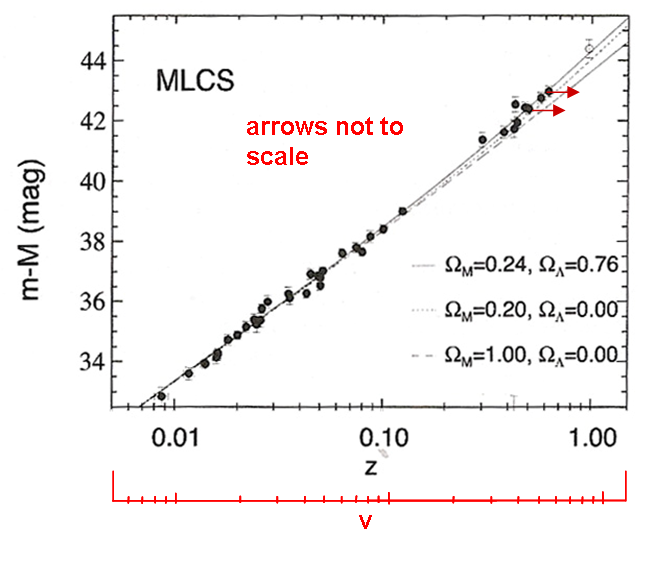Figure 3.3: Supernova 1a Hubble diagram - corrected

This means physically that the resulting acceleration vanishes.

3.4 An Alternative Explanation Using Inflation

Finally it shall be shown that also the classical inflation following the spatial ideas of Einstein can be used to explain the seeming acceleration effect.

It has, however, to be taken into account that this explanation needs the Basic Particle Model to explain the behaviour of elementary particles.

If we accept the idea of spatial inflation as shown in figure 2.1, then, at the earlier times which are represented in the upper right part of figure 1.1, space was smaller than today. So also all objects like the elementary particles have been necessarily smaller than today. This means that, if we assume that c was constant all the time as it is assumed by the understanding of Einstein, the rotational frequency within the elementary particles was greater than today. That means in turn that the frequencies of the atomic states have been greater than today and so also the frequencies of atomic transitions.

This assumption has the consequence that the speed related to the red shift observed was smaller in the past than generally assumed, and no recent acceleration has to be concluded.

4 Conclusion

The apparent acceleration of the universe at recent time can be explained as a measurement result based on incorrect assumptions. If we accept a variable c as assumed by a number of physicists during all times, it turns out to be a misinterpretation. And even if the understanding of ‘space’ as of Einstein is used, it turns out to be a measurement effect, supposed a proper particle model is assumed.

NOTE:

The concept of the Basic Particle Model was initially presented at the Spring Conference of the German Physical Society (Deutsche Physikalische Gesellschaft) on 24 March 2000 in Dresden by Dr. Albrecht Giese,
note@ag-physics.de

2019-11-23

 A.G.Riess et al 1998 Ap. J. 116:1009 ; "Observational evidence from supernovae for an accelerating universe and a cosmological constant" Astronomical  J.116: 1009–38.   10.1086/300499. http://www.arxiv.org/abs/astro-ph/9805201.

 A. Albrecht and J. Magueijo, Phys. Rev. D 59, 043516 (1999); A. Albrecht and J. Magueijo; "A time varying speed of light as a solution to cosmological puzzles" http://arxiv.org/abs/astro-ph/9811018;